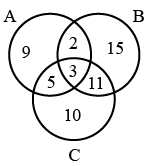Question 4

# In the given Venn diagram, ‘Group A’ represents those who can speak 'English’, ‘Group B’ represents those who can speak ‘Hindi’, and ‘Group C’ represents those who can speak ‘Marathi’. The numbers given in the diagram represent the number of persons in that particular category.How many persons can speak exactly two languages?

Solution

From the Venn diagram,

Number of persons who can speak exactly two languages = Intersection of A and B only + Intersection B and C only + Intersection of C and A only = 2 + 11 + 5 = 18

Hence, the correct answer is Option D